# HSSlive: Plus One & Plus Two Notes & Solutions for Kerala State Board

## BSEB Class 10 Maths Chapter 8 Introduction to Trigonometry Ex 8.1 Textbook Solutions PDF: Download Bihar Board STD 10th Maths Chapter 8 Introduction to Trigonometry Ex 8.1 Book AnswersBSEB Class 10 Maths Chapter 8 Introduction to Trigonometry Ex 8.1 Textbook Solutions PDF: Download Bihar Board STD 10th Maths Chapter 8 Introduction to Trigonometry Ex 8.1 Book Answers

BSEB Class 10th Maths Chapter 8 Introduction to Trigonometry Ex 8.1 Textbooks Solutions and answers for students are now available in pdf format. Bihar Board Class 10th Maths Chapter 8 Introduction to Trigonometry Ex 8.1 Book answers and solutions are one of the most important study materials for any student. The Bihar Board Class 10th Maths Chapter 8 Introduction to Trigonometry Ex 8.1 books are published by the Bihar Board Publishers. These Bihar Board Class 10th Maths Chapter 8 Introduction to Trigonometry Ex 8.1 textbooks are prepared by a group of expert faculty members. Students can download these BSEB STD 10th Maths Chapter 8 Introduction to Trigonometry Ex 8.1 book solutions pdf online from this page.

## Bihar Board Class 10th Maths Chapter 8 Introduction to Trigonometry Ex 8.1 Books Solutions

 Board BSEB Materials Textbook Solutions/Guide Format DOC/PDF Class 10th Subject Maths Chapter 8 Introduction to Trigonometry Ex 8.1 Chapters All Provider Hsslive

## How to download Bihar Board Class 10th Maths Chapter 8 Introduction to Trigonometry Ex 8.1 Textbook Solutions Answers PDF Online?

2. Click on the Bihar Board Class 10th Maths Chapter 8 Introduction to Trigonometry Ex 8.1 Answers.
3. Look for your Bihar Board STD 10th Maths Chapter 8 Introduction to Trigonometry Ex 8.1 Textbooks PDF.
4. Now download or read the Bihar Board Class 10th Maths Chapter 8 Introduction to Trigonometry Ex 8.1 Textbook Solutions for PDF Free.

## BSEB Class 10th Maths Chapter 8 Introduction to Trigonometry Ex 8.1 Textbooks Solutions with Answer PDF Download

Find below the list of all BSEB Class 10th Maths Chapter 8 Introduction to Trigonometry Ex 8.1 Textbook Solutions for PDF’s for you to download and prepare for the upcoming exams:

## BSEB Bihar Board Class 10th Maths Solutions Chapter 8 Introduction to Trigonometry Ex 8.1

Question 1.
In ∆ ABC, right angled at B, AB = 24 cm, BC = 7 cm. Determine :
(i) sin A, cos A (ii) sin C, cos C
Solution:
Let us draw a right ∆ ABC.By using the Pythagoras theorem, we have :
AC² = AB² + BC² = (24)² + (7)²
= 576 + 49 = 625
So, AC = 625‾‾‾‾√ cm = 25 cm
(i) sin A = 𝐵𝐶𝐴𝐶 = 725, cos A = 𝐴𝐵𝐴𝐶 = 2425
(ii) sin C = 𝐴𝐵𝐴𝐶 = 2425, cos C = 𝐵𝐶𝐴𝐶 = 725

Question 2.
In adjoining figure, find tan P – cot R.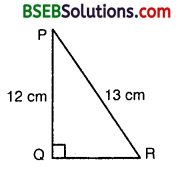Solution:
By using the Pythagoras theorem, we have :
PR² = PQ² + QR² or 13² = 12² + QR²
or QR² = 13² – 12² = 169 – 144 = 25
So, QR = 25‾‾‾√ cm = 5 cm
∴ tan P = 𝑄𝑃𝑃𝑄 = 512 and cot R = 𝑄𝑅𝑃𝑄 = 512
Hence, tan P – cot R = 512 – 512 = 0.

Question 3.
If sin A = 34, calculate cos A and tan A.
Solution:
Consider a ∆ ABC in which ∠B = 90°.For ∠A, we have :
Perp. (opposite side) = BC
and Hyp. = AC.Question 4.
Given 15 cot A =8, find sin A and sec A.
Solution:
Consider a ∆ ABC in which ∠B = 90°.
For ∠A, we have:
Base AB, Perp. = BC and Hyp. = AC.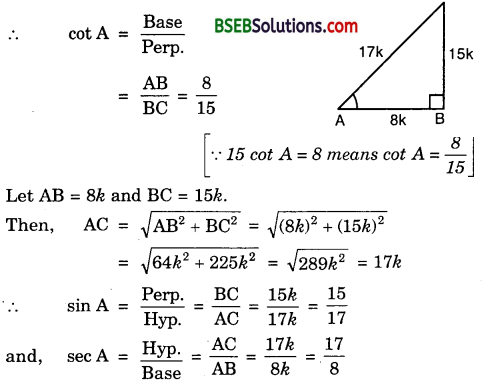Question 5.
Given sec θ = 1312, calculate all other trigonometric ratios.
Solution:
Consider a ∆ ABC in which ∠A = 0° and ∠B = 90° Then, Base = AB, Perp. = BC and Hyp. = AC.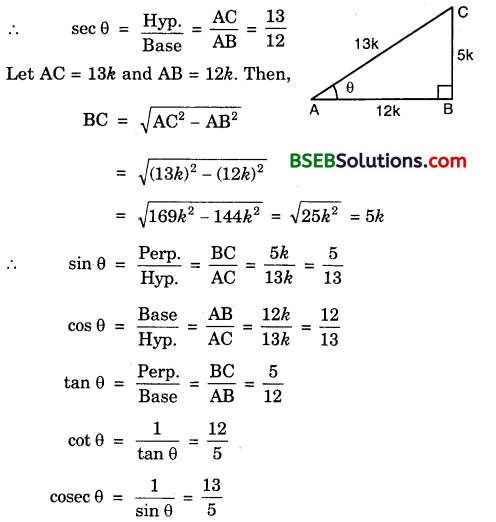Question 6.
If ∠A and ∠B are acute angles such that cos A = cos B, then show that ∠A = ∠B.
Solution:
Let us consider two right triangles PQA and RSB in which cos A = cos B (see the figure).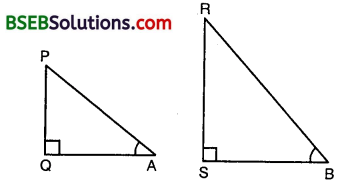We have:
cos A = 𝑄𝐴𝑃𝐴
and cos B = 𝑆𝐵𝑅𝐵
Thus, it is given that
𝑄𝐴𝑃𝐴 = 𝑆𝐵𝑅𝐵
so, 𝑄𝐴𝑆𝐵 = 𝑃𝐴𝑅𝐵 = K(say) … (1)
Now, by Pythagoras Theorem,Therefore, from (1) and (2), we have
𝑄𝐴𝑆𝐵 = 𝑃𝐴𝑅𝐵 = 𝑃𝑄𝑅𝑆
Hence, ∆ PQA ~ ∆ RSB [SSS similarity]
Therefore, ∠A = ∠B [Corresponding angles]

Question 7.
If cot θ = 78, evaluate :
(i) (1+sin𝜃)(1−sin𝜃)(1+cos𝜃)(1−cos𝜃)
(ii) cot² θ
Solution:
Consider a ∆ ABC in which ∠A = 0 and ∠B = 90°.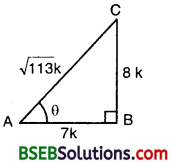Then, Base = AB, Perp = BC and Hyp = AC
∴ cot θ = 𝐵𝑎𝑠𝑒𝑃𝑒𝑟𝑝 = 𝐴𝐵𝐵𝐶 = 78
Let AB = 7k and BC = 8k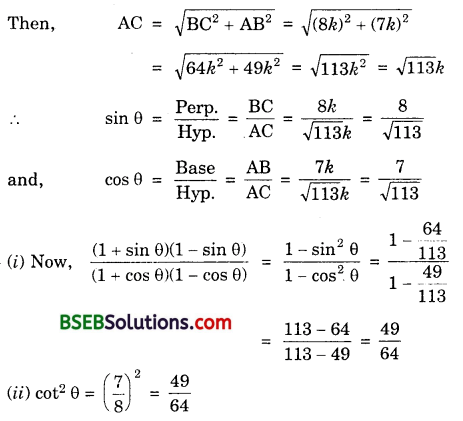Question 8.
If 3 cot A = 4, check whether 1−tan2𝐴1+tan2𝐴 = cos² A – sin² A or not.
Solution:
Consider a ∆ ABC in which ∠B = 90°.
Foe ∠A, we have:Base = AB, Perp = BC and Hyp = AC
∴ cot A = 𝐵𝑎𝑠𝑒𝑃𝑒𝑟𝑝 = 𝐴𝐵𝐵𝐶 = 43
Let AB = 4k and BC = 3k [3 cot A = 4 ⇒ cot A = 43 ]Question 9.
In ∆ ABC right angled at B, if tan A = 13√, find the value of
(i) sin A cos C + cos A sin C
(ii) cos A cos C – sin A sin C
Solution:
Consider a ∆ ABC, in which ∠B = 90°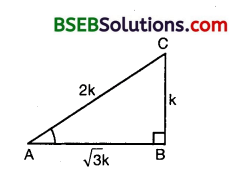For ∠A, we have :
Base = AB,
Perp. = BC .
and Hyp. = AC
∴ tan A = 𝑃𝑒𝑟𝑝𝐵𝑎𝑠𝑒 = 𝐵𝐶𝐴𝐵 = 13√
Let BC = k and AB = 3‾√k.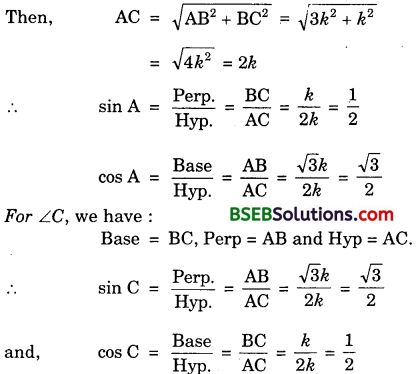(i) sin A cos C + cos A sin C = 12 x 12 + 3√2 x 3√2
= 14 x 34 = 44 = 1
(ii) cos A cos C – sin A sin C = 3√2 x 12 – 12 3√2 = 0

Question 10
In ∆ PQR, right angled at Q, PR + QR = 25 cm and PQ = 5 cm. Determine the values of sin P, cos P and tan P.
Solution:
In ∆ PQR, right ∠d at Q,PR + QR = 25 cm and PQ = 5 cm.
Let QR = x cm
∴ PR = (25 – x) cm
By Pythagoras theorem, we have :
NJ). IUIJY MA’l’
RP² = RQ² + QP²
So, (25 – x)² = x² + 5²
or 625 – 50x + x² = x² + 25
or – 50x = – 600 or x = −600−50 = 12
∴ RQ = 12cm
So, RP = (25 – 12)cm = 13 cm
Now, sin P = 𝑅𝑄𝑅𝑃 = 1213
cos P = 𝑃𝑄𝑅𝑃 = 513
and, tan P = 𝑅𝑄𝑃𝑄 = 125

Question 11.

1. The value of tan A is always less than 1.
2. sec A = 125 for some value of angle A.
3. cos A is the abbreviation used for the cosecant of angle A.
4. cot A is the product of cot and A.
5. sin θ = 43 for some angle θ.

Solution:

1. False because sides of a right triangle may have any length, so tan A may have any value.
2. True as sec A is always greater than or equal to 1.
3. False as cos A is the abbreviation used for cosine A.
4. False as cot A is not the product of ‘cot’ and A. ‘cot separated from A has no meaning.
5. False as sin 0 cannot be > 1.

## Bihar Board Class 10th Maths Chapter 8 Introduction to Trigonometry Ex 8.1 Textbooks for Exam Preparations

Bihar Board Class 10th Maths Chapter 8 Introduction to Trigonometry Ex 8.1 Textbook Solutions can be of great help in your Bihar Board Class 10th Maths Chapter 8 Introduction to Trigonometry Ex 8.1 exam preparation. The BSEB STD 10th Maths Chapter 8 Introduction to Trigonometry Ex 8.1 Textbooks study material, used with the English medium textbooks, can help you complete the entire Class 10th Maths Chapter 8 Introduction to Trigonometry Ex 8.1 Books State Board syllabus with maximum efficiency.

## FAQs Regarding Bihar Board Class 10th Maths Chapter 8 Introduction to Trigonometry Ex 8.1 Textbook Solutions

#### How to get BSEB Class 10th Maths Chapter 8 Introduction to Trigonometry Ex 8.1 Textbook Answers??

Students can download the Bihar Board Class 10 Maths Chapter 8 Introduction to Trigonometry Ex 8.1 Answers PDF from the links provided above.

#### Can we get a Bihar Board Book PDF for all Classes?

Yes you can get Bihar Board Text Book PDF for all classes using the links provided in the above article.

## Important Terms

Bihar Board Class 10th Maths Chapter 8 Introduction to Trigonometry Ex 8.1, BSEB Class 10th Maths Chapter 8 Introduction to Trigonometry Ex 8.1 Textbooks, Bihar Board Class 10th Maths Chapter 8 Introduction to Trigonometry Ex 8.1, Bihar Board Class 10th Maths Chapter 8 Introduction to Trigonometry Ex 8.1 Textbook solutions, BSEB Class 10th Maths Chapter 8 Introduction to Trigonometry Ex 8.1 Textbooks Solutions, Bihar Board STD 10th Maths Chapter 8 Introduction to Trigonometry Ex 8.1, BSEB STD 10th Maths Chapter 8 Introduction to Trigonometry Ex 8.1 Textbooks, Bihar Board STD 10th Maths Chapter 8 Introduction to Trigonometry Ex 8.1, Bihar Board STD 10th Maths Chapter 8 Introduction to Trigonometry Ex 8.1 Textbook solutions, BSEB STD 10th Maths Chapter 8 Introduction to Trigonometry Ex 8.1 Textbooks Solutions,
Share: